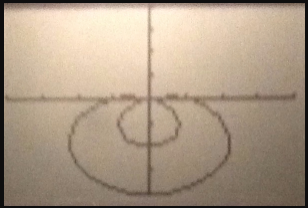# Use the polar symmetry tests to determine if the graph is symmetric

Use the polar symmetry tests to determine if the graph is symmetric about the x-axis, the y-axis, or the origin. Support your algebraic solution with a grapher.
r=1−3sinθ